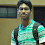# 3 Ways to Measure Execution Time in R with Examples

In R, there are multiple ways to measure the running time of your R Code.

## Method 1: Using the `Sys.time()` function

To measure the execution time of R code, we can use the Sys.time function. Put it before and after the code and take difference of it to get the execution time of code.

```start.time <- Sys.time()

result <- sum(1:10000)

end.time <- Sys.time()
time.taken <- round(end.time - start.time,2)
time.taken
```

Result : Time difference of 1.12 secs

## Method 2: Using the `microbenchmark` package

The microbenchmark package allows you to accurately measure the execution time of small code snippets, especially when you need to compare different approaches or functions. You can install the package by using command `install.packages("microbenchmark")`

Let's take a simple example to measure the running time of two different approaches for calculating the sum of numbers from 1 to N.

Approach 1: Using a loop
Approach 2: Using the sum() function.

```# Load the microbenchmark package
library(microbenchmark)

# Function to calculate the sum of numbers from 1 to N using a loop
sum_using_loop <- function(N) {
result <- 0
for (i in 1:N) {
result <- result + i
}
return(result)
}

# Function to calculate the sum of numbers from 1 to N using the sum() function
sum_using_sum <- function(N) {
return(sum(1:N))
}

# Measure the running time using microbenchmark
result <- microbenchmark(
sum_using_loop(10000),
sum_using_sum(10000),
times = 100
)

print(result)
```

The times parameter sets how many times each function is executed to get a more accurate measurement of the execution time.

See the output below. sum_using_sum() function is significantly faster than the sum_using_loop() function.

```                  expr   min     lq    mean median    uq    max neval
sum_using_loop(10000) 522.0 566.15 630.333  571.0 600.9 5881.4   100
sum_using_sum(10000)   1.3   1.40  36.513    1.5   1.6 3399.9   100
```

## Method 3: Using the `rbenchmark` package

The rbenchmark package is another option for benchmarking your R code. It's useful when you want to compare the performance of different functions or versions of your code. You can install the package by using command `install.packages("rbenchmark")`

```# Load the rbenchmark package
library(rbenchmark)

# Function to calculate the sum of numbers from 1 to N using a loop
sum_using_loop <- function(N) {
result <- 0
for (i in 1:N) {
result <- result + i
}
return(result)
}

# Function to calculate the sum of numbers from 1 to N using the sum() function
sum_using_sum <- function(N) {
return(sum(1:N))
}

# Measure the running time using benchmark
result <- benchmark(
sum_using_loop(10000),
sum_using_sum(10000),
replications  = 100
)

print(result)
```

The replications parameter inside the functions determines how many times each function is executed during the benchmarking process.

Output:
```                   test replications elapsed relative user.self sys.self
1 sum_using_loop(10000)          100    0.06        6      0.05        0
2  sum_using_sum(10000)          100    0.01        1      0.01        0
```
Related Posts
ShareDeepanshu founded ListenData with a simple objective - Make analytics easy to understand and follow. He has over 10 years of experience in data science. During his tenure, he worked with global clients in various domains like Banking, Insurance, Private Equity, Telecom and HR.

2 Responses to "3 Ways to Measure Execution Time in R with Examples"
1.I have a doubt. I'm writing R code as a function statement. for example:

return(df)
}

I need to measure the complation time with the date. can i use your start.time and end.time within your function, if so, can you please explain?. or any other ways to do that.

1.Check out this code -